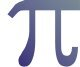Free Fun Math Facts and Activities - MyHomeSchoolMath

myhomeschoolmath.comTitle
Go to contentFree Fun Math Facts and Activities

Free Fun Math Facts and Activities
Here you can find lots of fun math facts you can share with your friends or class... Quess the number. Magic number. Multiplying in an easy way. Roman, Greek, Egiptian Numerals. Prime Numbers. Large Numbers. Calculate the day. How old are you exactly?
Quess the number
Cut the paper and have fun with your friends. You can quess the number which they imagine. Free PDF here!
Adding more numbers in a series
Ask someone to add all the numbers from 1 to 200 and then surprise them by giving them the result in less than one minute. How?
The sum of the first and last, second and second last numbers and so on are always the same. Meaning, 1+200, 2+199, 3+198 is always 201. In total we have 100 sums like this so 201*100 = 20 100.
Of course, you can also ask for the sum of numbers from 1 to 100 or 101 to 300.
Multiplication table with the help of fingers
Do you know that you can multiply numbers bigger than 5 with the help of your fingers. But, that is just to assist you, you should know the multiplication table by heart. Free PDF here!
Multiplying numbers from 11 to 20 in an easy way
Two double-digit numbers smaller than 20 can be multiplied in a shorter and quicker way. Free PDF here!
How to quickly multiply number 11 with double-digit numbers?
Number 11 and any double-digit number can be multiplied in a shorter and quicker way. Free PDF here!
How is this possible?
We have four shapes and we create a triangle. If we assemble the shapes in a different way on the same surface we will have a “hole” ? Free PDF here!
Calculate the day
A formula that can help us calculate what day any date was. Free PDF here!
Roman, Greek, Egiptian Numerals
Here you can find the chart of Roman, Greek and Egiptian numerals.
Roman numerals are a system of numerical notations used by the old Romans. Greek numerals are a bit more complicated than Roman. These Acrophonic Greek numerals were used in ancient Greece up to Roman times (100 BC). The system of ancient Egyptian numerals was used in Ancient Egypt around 3000 BC until the early first millennium AD, and written in hieroglyphs. Free PDF here!
Prime Numbers
Here you can find the first 500 prime numbers. Free PDF here!
Multiples
Some interesting results of multiplication. Free PDF here!
Large numbers
What is the largest number that you know? A million? A milliard? A billion? And after that? Check to see to what number you can count to. Free PDF here!
Sum = 100
How can we get a sum of 100 by adding numbers til 9, ordering according to their size. Free PDF here!
Magic number
The remainder that we get when we divide the number 2519 with numbers from 1 to 10. Free PDF here!
The PI Number
Probably the most popular number in human history. The Pi Number is usually written as 3.14, but in reality it has an infinite number of decimal places. The simplest way to describe the Pi Number is that is a number which gives us the ratio of the circle’s circumference and its diameter. There were many attempts throughout history to get the Pi Number to as many decimals as possible – and here is the Pi Number calculated to 115 decimal places:3.14159 26535 89793 23846 26433 83279 50288 41971 69399 37510 58209 74944 59230 78164 06286 20899 86280 34825 34211 70679 82148 08651 32823...
Same from both sides
Read, calculate, turn over and try again. It is the same, isn't it? Free PDF here!
Back to content